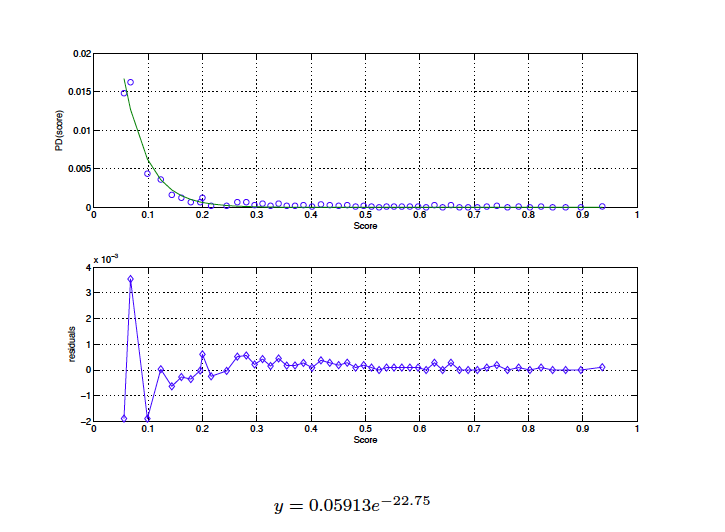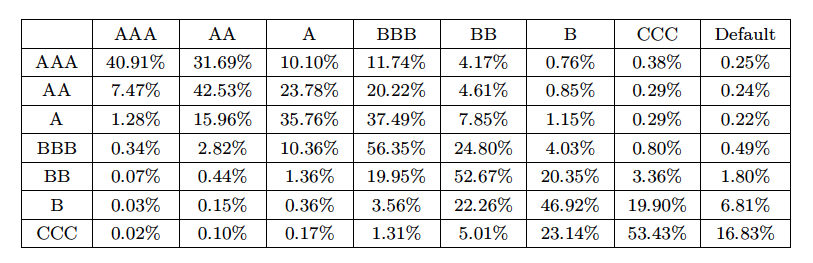• ITA
Category: Credit Rating Methodologies

# How to check if a rating model is valid [Part 2]

## Today we continue the study of the methods that should be used to understand if a rating model is appropriate or not.

The last time we had seen and analyzed the first two points that a model should possess:

• an adequate distribution of failures between rating categories;
• a high discriminatory power;

Now we conclude the analysis of the remaining key features:

• a comparison between the theoretical probability of default and rates actual default;
• adequate matrices migration and consequent cumulative default rates triennial.

### Comparability between the theoretical probability of default and actual default rates.

Often for methods of credit scoring the theoretical probability of default is formed by the application of a mathematical formula: following an optimization process which aims to minimize an error function (or a likelihood function) is obtained by the so-called “Probability of default” or PD.
The values ​​of PD must not only be validated but, through a complicated process of calibration, they must be modified so that they can express the actual default frequency of the sample studied. This process is often complicated and not without pitfalls.

Since the probability of default associated with the score (hereinafter PD (score)) must meet two basic requirements: be monotonous and satisfy the constraint 0 ≤ ≤ 1 PD, we generally prefer to use a function exponential of the form y = aXe^(bx).
The steps to be followed for the determination PD (score), are the following:

1. build a panel containing the score associated with each company and a dichotomous variable (0 or 1) indicating whether the company in question is failed or is healthy;
2. divide the population into buckets, each of which containing a equal number of observations (for example, 2%);
3. every score (the average in the bucket) is associated with a default rate (DF) obtained as the ratio between the number of companies failed and that of Companies solvents;
4. shows the results in a scatter plot, so we proceed to determine the better function interpolating the data.

The estimate of the free parameters a and b must be conducted through a optimization to minimize an error function; generally using the method of least squares.

Below is an example of calibration:### Transition Matrices calibration

As stated in the requirements which ECAIs should possess, migration matrices play an important role; more precisely it notes that “agencies must still possess historical data covering the annual rates of default – theoretical, where available, and effective – and matrices transition with a temporal depth of at least 5 years.
They must also be calculated cumulative default rates in three years reported on the basis of such series.
Compliance with this requirement is checked even temporal in relation to the size of the cohorts for different rating classes in each year. ”
For transition or migration is defined as the change in the credit of a counterparts. We shall, therefore, by estimating the probability of migration from one rating class to another; these probabilities are represented in a transition matrix M = [mij] of dimension (SxS), where s is the number of rating classes (including the class D = default). The probability quantifies mij the probability that during a certain time horizon (typically a year), a counterpart to migrate from the rating to rating j. The properties of M can be summarized as follows:

• its elements are non-negative;
• the sum of the elements on the i-th row is equal to one;
• the last column contains the PD on the time horizon considered;
• the default is considered to be an absorbing state, that is the last line the matrix is ​​equal to mis = PDi;
• if M is the transition matrix relating to a period of one year, the transition matrix relating to a period of years n is given by Mn = Mn.

But because they are of such great importance matrices migration?

Because from their observation can be drawn two basic information:

• the probability that a company maintains its rating year after year (values ​​on the diagonal);
• the probability that a company should be technically in default (ie get a rating that is lower than the distressed CCC).

Example of matrix migration:In these last two posts we have seen as a rating model must be validated. It is essential to know these methods because only applying them efficiently you can give assurance to customers that the assessments are really accurate!